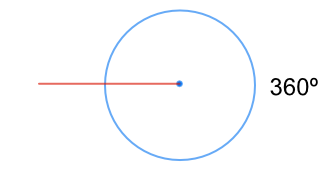Angles at a Point

# Angles at a Point

GCSE(F),

Angles about a point add up to 360º (which is a complete circle).If a point is on a straight line, then the angles on each side of the line add up to 180º.## Examples

1. What is the value of the angle A in this diagram?